# Two Sample t Test with JMP

## What is Two Sample t Test?

Two sample t test is a hypothesis test to study whether there is a statistically significant difference between the means of two populations.

• Null Hypothesis (H0): μ1 = μ2
• Alternative Hypothesis Ha) : μ1μ2

Where: μ1 is the mean of one population and μ2 is the mean of the other population of our interest.

#### Assumptions of Two Sample T Tests

• The sample data drawn from both populations are unbiased and representative
• The data of both populations are continuous
• The data of both populations are normally distributed
• The variances of both populations are unknown
• Two sample t-test is more robust than a z-test when the sample size is small (< 30)

#### Three Types of Two Sample T Tests

1. Two sample t test when the variances of two populations are unknown but equal
Two sample t test (when σ21 = σ22)
2. Two sample t test when the variances of the two population are unknown and unequal
Two sample t test (when σ21 ≠ σ22)
3. Paired t test when the two populations are dependent of each other, so each data point from one distribution corresponds to a data point in the other distribution.

### Test of Equal Variance

To check whether the variances of two populations of interest are statistically significant different, we use the test of equal variance.

• Null Hypothesis (H0): σ21 = σ22
• Alternative Hypothesis (Ha): σ21 ≠ σ22

An F-test is used to test the equality of variances between two normally distributed populations.
An F-test is a statistic hypothesis test in which the test statistic follows an F-distribution when the null hypothesis is true. The most known F-test is the test of equal variance for two normally distributed populations. The F-test is very sensitive to non-normality. When any one of the two populations is not normal, we use the Brown–Forsythe test to check the equality of variances.

### Decision Rules of a Two Sample T Test

• Null Hypothesis (H0): μ1 = μ2
• Alternative Hypothesis(Ha) : μ1μ2

If |tcalc| > tcrit, we reject the null and claim there is a statistically significant difference between the means of the two populations.
If |tcalc| < tcrit, we fail to reject the null and claim there is not any statistically significant difference between the means of the two populations.

### Use JMP to Run a Two-Sample T-Test

Case study: We are trying to compare the average retail price of a product in state A and state B.
Data File: “TwoSampleT-Test.jmp”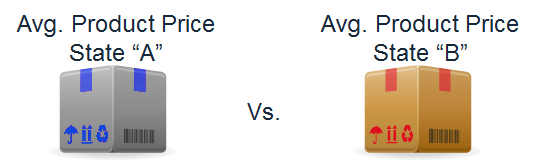•  Null Hypothesis (H0): μ1 = μ2
• Alternative Hypothesis(Ha) : μ1μ

In this example, we will compare a product's average price in two different states. The null hypothesis is that the price in state A equals that in state B.

Step 1: Test the normality of the retail price for both state A and B.

1. Click Analyze -> Distribution
2. Select “Retail Price” as “Y, Columns”
3. Select “State” as “By”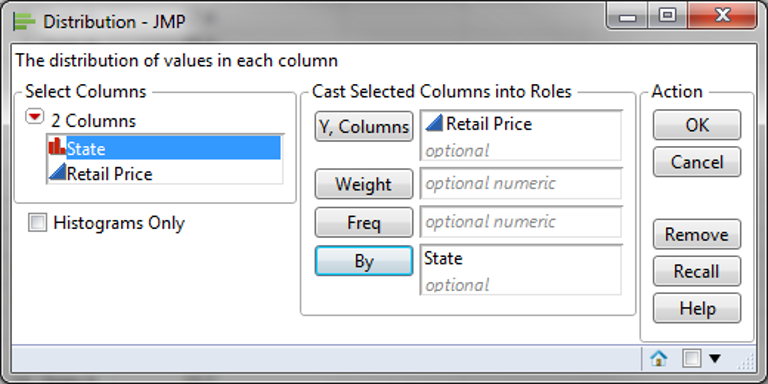4. Click “OK”
5. Click on the red triangle button next to “Retail Price” in the Distribution page for “State = State A”
6. Click Continuous Fit -> Normal
7. Click on the red triangle button next to “Fitted Normal”
8. Select “Goodness of Fit”
9. Repeat the same to test the normality for “State = State B”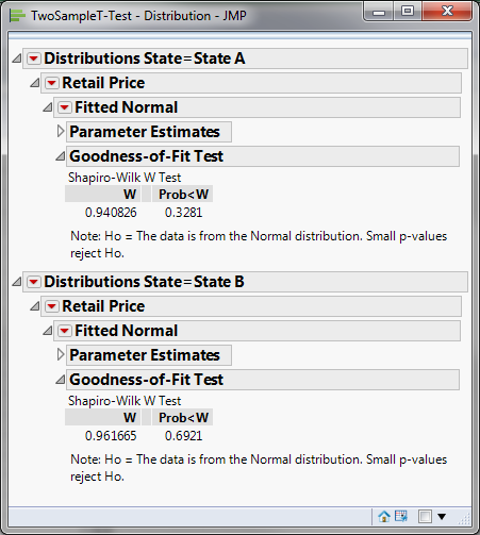• Null Hypothesis(H0): The data are normally distributed
• Alternative Hypothesis(Ha): The data are not normally distributed

Both retail price data of state A and B are normally distributed since the p-values are both greater than alpha level (0.05). If any data series is not normally distributed, we need to use hypothesis testing methods other than the two sample t-test.

Following the instructions on the previous page, we determine if the data follow a normal distribution. In this case, you can see that the p-value for both is higher than 0.05, so we fail to reject the null hypothesis that the data are normally distributed. We must use a different test if the data are not normally distributed.

Step 2: Test whether the variances of the two data sets are equal.

• Null Hypothesis(H0):
• Alternative Hypothesis(Ha):
1. Click Analyze -> Fit Y by X
2. Select “Retail Price” as “Y, Response”
3. Select “State” as “X, Factor”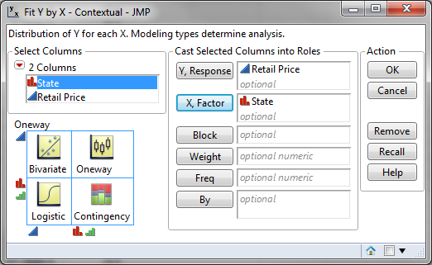4. Click “OK”
5. Click on the red triangle button next to “One-Way Analysis of Retail Price by State”
6. Select “Unequal Variances”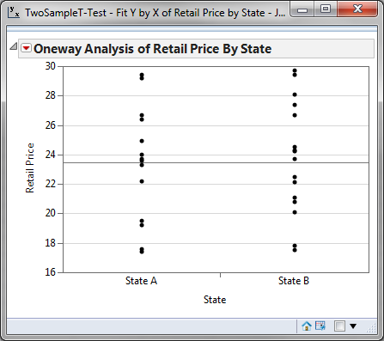Because the retail prices at state A and state B are both normally distributed, an F test is used to test their variance equality. The p-value of F test is 0.870, greater than the alpha level (0.05), so we fail to reject the null hypothesis, and we claim that the variances of the two data sets are equal. We will use the two sample t-test (when σ21 = σ22) to compare the means of the two groups. If σ21 ≠ σ22, we will use the two sample t-test (when σ21σ22) to compare the means of the two groups.

Step 3: Run two-sample t-test to compare the means of two groups.

1. Click on the red triangle button next to “One-Way Analysis of Retail Price By State”
2. Select “Means/Anova/Pooled t”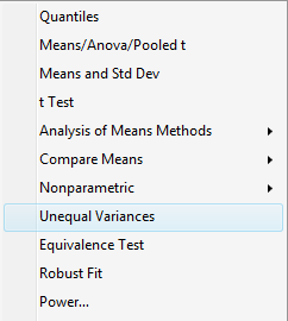Since the p-value of the t-test (assuming equal variance) is 0.665, greater than the alpha level (0.05), we fail to reject the null hypothesis, and we claim that the means of the two data sets are equal. If the variances of the two groups are not equal, we will need to use the two-sample t-test (when σ1σ2) to compare the means of the two groups.

• Click on the red triangle button next to “One-Way Analysis of Retail Price By State”
• Select “t test”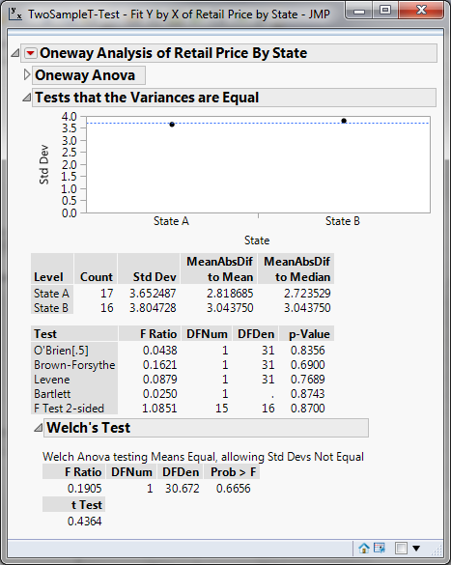Model summary: Since the p-value of the t-test (assuming unequal variance) is 0.666, greater than the alpha level (0.05), we fail to reject the null hypothesis, and we claim that the means of the two groups are equal.